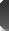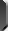Search :>> Products SeriesBall Valve Series Check Valve Series Globe Valve Series Gate Valve Series Strainer Series Bellows Series
 Ball Valve Series Check Valve Series Globe Valve Series Gate Valve Series Strainer Series>> Contact infomationADDRESS:Baoxi road ,Dongou industrial park of Wenzhou Oubei POPďĽš325102 TELďĽš0086-577-67371611 21811188 FAXďĽš0086-577- 67371612 WEBďĽšhttp://www.kz-valve.com EMAILďĽškz@kz-valve.com>> News > Common units conversion tableCommon units conversion table
DataTimeďĽš2008/3/10 10:45:38
From To ďĽš ---
 Length 1 kilometres (km) = 0.621 miles (mile) 1 metre (m) = 3.281 feet (ft) = 1.094 yards (yd) 1 centimeter (cm) = 0.394 inches (in) 1 mile (mile) = 1.609 kilometers (km) 1 feet (ft) = 0.3048 meters (m) 1-inch (in) = 2.54 centimeters (cm) 1 mile (n mile) = 1.852 kilometers (km) 1 yards (yd) = 0.9144 meters (m) one feet (ft) = 12 inches (in) 1 yards (yd) = 3 feet (ft) 1 mile (mile) = 5,280 feet (ft) 1 nautical miles (n mile) = 1.1516 miles (mile)         Quality 1 metric ton (t) = 1,000 kilograms (kg) = 2205 pounds (lb) = 1.102 short ton (sh.ton) = 0.934 tonnes (long.ton) 1 kilograms (kg) = 2.205 pounds (lb) a short ton ( sh.ton) = 0.907 tons (t) = 2,000 pounds (1 b) A long tons (long.ton) = 1.016 tons (t) 1 pound (lb) = 0.454 kilograms (kg) 1 ounces (oz) = 28.350 grams (g)           Density 1 kg / m 3 (kg / m 3) = 0.001 g / cm 3 (g / cm 3) = 0.0624 lb / ft 3 (lb / ft 3) 1 lb / ft 3 (lb / ft 3) = 16.02 kg / m 3 (kg / m 3) 1 lb / inch (lb / in 3) = 27679.9 kg / m 3 (kg / m 3) 1 lb / US gallon (lb / gal) = 119.826 kg / m 3 (kg / m 3) 1 lb / British gallon (lb / gal) = 99.776 kg / m 3 (kg / m 3) 1 lb / (oil) barrels (lb / bbl) = 2.853 kg / m 3 (kg / m 3) Baume density 1 = 140/15.5 ˇć, the proportion of API = -130 ˇć 141.5/15.5 the proportion -131.5         Pressure 1 MPa (MPa) = 145 lb / 2 inch (psi) = 10.2 kg / cm 2 (kg / cm 2) = 10 bar (bar) = 9.8 atm (at m) 1 lb / 2 inch (psi) = 0.006895 MPa (MPa) = 0.0703 kg / cm 2 (kg / cm 2) = 0.0689 Pakistan (bar) = 0.068 atm (at m) 1 bar (bar) = 0.1 MPa (MPa) = 14.503 lb / 2 inch (psi) = 1.0197 kg / cm 2 (kg / cm 2 ) = 0.987 atm (at m) 1 atm (at m) = 0.101325 MPa (MPa) = 14.696 lb / 2 inch (psi) = 1.0333 kg / cm 2 (kg / cm 2) = 1.0133 Pakistan (bar)         Area One square km (km 2) = 100 hectares (ha) = 247.1 Acres (acre) = 0.386 square miles (2 mile) 1 square metres (m 2) = 10.764 square feet (ft 2) 1 hectares (acre) = 100 square metres (m 2) 1 hectare (ha) = 10,000 square meters (m 2) = 2.471 acres (acre) 1 square mile (2 mile) = 2.590 square kilometers (km 2) 1 acres (acre) = 0.4047 hectares (ha) = 40.47 * 10 -3 square kilometres (km 2) = 4,047 square meters (m 2) One square feet (ft 2) = 0.093 square meters (m 2) 1 square inches (in 2) = 6.452 square centimeters (cm 2) one square yards (yd 2) = 0.8361 square meters (m 2)         Volume 1 cubic meters (m 3) = 1,000 liters (liter) = 35.315 cubic feet (ft 3) = 6.290 barrels (bbl) 1 cubic feet (ft 3) = 0.0283 cubic meters (m 3) = 28.317 liters (l) a 1000 cubic feet (mcf) = 28.317 cubic meters (m 3) 1 million cubic feet (MMcf) = 2.8317 million cubic meters (m 3) 1 billion cubic feet (bcf) = 28.317 million cubic meters (m 3) 1 trillion cubic feet (tcf) = 28.317 billion cubic meters (m 3) 1 cubic inches (in 3) = 16.3871 cubic centimeters (cm 3) 1 acres feet = 1,234 cubic meters (m 3) a barrel (bbl) = 0.159 cubic meters (m 3) = 42 US gallons (gal) 1 US gallon (gal) = 3.785 liters (l) United States 1 quart (qt) = 0.946 liters (l) 1 US pint (pt) = 0.473 liters (l) 1 US Kyrgyzstan ears (gi) = 0.118 liters (l) a British gallon (gal) = 4.546 liters (l)           Kinematic viscosity 1 ft / s (2 ft / s) = 9.29030 * 10 -2 M 2 / s (2 m / s) 1 Andean (St) = 10 -4 m 2 / s (2 m / s) 1% Andean (eSt) = 10 -6 m 2 / s (2 m / s) = 1 mm 2 / s (mm 2 / s)         Dynamic viscosity 1 Mooring (P) = 0.1 Pago seconds (Pa s) 1% Mooring (cP) = 10 -3 Pago seconds (Pa s) 1 1000 Caledon seconds / m 2 = 9.80505 Pago seconds (Pa s) 1 lb of seconds / ft 2 (1bf s / ft 2) = Pago 47.8803 seconds (Pa s)         Of 1 Newton (N) = 0.225 pounds of (1 bf) = 0.102 1000 Caledon (kgf) 1 1000 Caledon (kgf) = 9.81 Newton (N) one pounds of (1 bf) = 4.45 Newton (N) 1 dyne (dyn) = 10 -5 Newton (N)         Temperature K (degrees Kelvin) = 5 / 9 (¨H +459.67) K = n ¨H ˇć +273.15 = [(n-32) * 5 / 9] ˇć N ˇć (C) = (5 / 9 n +32) 1 ¨H ¨H (F) = 5 / 9 ˇć (temperature difference)         Heat transfer coefficient 1 kcal (m 2:00 ˇć) [kcal / (m 2 h ˇć)] = 1.6279 watts / (m 2 Kelvin) [W (K m 2)] 1 British thermal units / (ft 2 When ¨H) [Btu / (h ¨H ft 2)] = 5.67826 watts / (m 2 Kelvin) [W (K m 2)] 1 m 2:00 ˇć / kilocalories (m 2 h ˇć / kcal) = 0.86000 m 2 Kelvin / watts (m 2 K / W) 1 kcal (m 2) (kcal / h m 2) = 1.16279 watts / m 2 (W / m 2)         Thermal Conductivity 1 kcal (m 2:00 ˇć) [kcal / (m 2 h ˇć)] = 1.16279 watts / (m Kelvin) [W (m K)] 1 British thermal units / (ft 2:00 ¨H) [Btu / (h ¨H ft 2)] = 1.7303 watts / (m Kelvin) [W (m K)]         Hematocrit heat 1 kcal / (kg ˇć) [kcal / (kg ˇć)] = 1 British thermal units / (pounds ¨H) [Btu / (lb ¨H)] = 4186.8 J / (kg Kelvin) [J / (kg K)]         Thermal power 1 Joule = 0.10204 1000 Kemi 10 -7 = 2.778 * 3.777 * kWh = 10 -7 metric horsepower hour = 3.723 * 10 -7 inch horsepower hour = 2.389 * 10 -4 kcal = 9.48 * 10 -4 British thermal Unit 1 card (cal) = 4.1868 joules (J) 1 British thermal units (Btu) = 1055.06 joules (J) 1 1000 Caledon metres (kgf m) = 9.80665 joules (J) one feet of pounds (ft 1bt) = 1.35582 joules (J) 1-meter-horsepower hour (hp h) = 2.64779 * 10 6 joules (J) An English-horsepower hour (UKHp h) = 2.68452 * 10 6 joules (J) one kilowatt hours (kw h) = 3.6 * 10 6 joules (J) 1 kcal = 4186.75 joules (J)         Power 1000 Caledon 1 m / s (kgf m / s) = 9.80665 watts (W) 1-meter-horsepower (hp) = 735.499 watts (W) 1 card / s (cal / s) = 4.1868 watts (W) 1 British thermal units / hour (Btu / h) = 0.293071 watts (W)         Speed 1 ft / sec (ft / s) = 0.3048 meters / second (m / s) 1 miles / hour (mile / h) = 0.44704 meters / second (m / s)         Penetration 1 = 1000 cents Darcy Darcy 1 cm2 (cm 2) = 9.81 * 10 7 Darcy